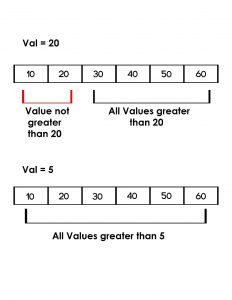Open In App

# Python | Check if all the values in a list that are greater than a given value

Given a list, print all the values in a list that are greater than the given valueExamples:

```Input : list = [10, 20, 30, 40, 50]
given value = 20
Output : No

Input : list = [10, 20, 30, 40, 50]
given value = 5
Output : Yes```

Method 1: Traversal of list

By traversing in the list, we can compare every element and check if all the elements in the given list are greater than the given value or not.

Implementation:

## Python

 `# python program to check if all``# values in the list are greater``# than val using traversal` `def` `check(list1, val):``    ` `    ``# traverse in the list``    ``for` `x ``in` `list1:` `        ``# compare with all the values``        ``# with val``        ``if` `val>``=` `x:``            ``return` `False``    ``return` `True``    ` `    ` `# driver code``list1 ``=``[``10``, ``20``, ``30``, ``40``, ``50``, ``60``]``val ``=` `5``if``(check(list1, val)):``    ``print``"Yes"``else``:``    ``print``"No"` `val ``=` `20``if``(check(list1, val)):``    ``print``"Yes"``else``:``    ``print``"No"`

Output

```Yes
No```

Time Complexity: O(n)
Auxiliary Space: O(1)

Method 2: Using all() function:

Using all() function we can check if all values are greater than any given value in a single line. It returns true if the given condition inside the all() function is true for all values, else it returns false.

Implementation:

## Python

 `# python program to check if all``# values in the list are greater``# than val using all() function` `def` `check(list1, val):``    ``return``(``all``(x > val ``for` `x ``in` `list1))``    ` `    ` `# driver code``list1 ``=``[``10``, ``20``, ``30``, ``40``, ``50``, ``60``]``val ``=` `5``if``(check(list1, val)):``    ``print``"Yes"``else``:``    ``print``"No"` `val ``=` `20``if` `(check(list1, val)):``    ``print``"Yes"``else``:``    ``print``"No"`

Output

```Yes
No```

Time Complexity: O(n)
Auxiliary Space: O(1)

Method 3 : Using min() method

## Python3

 `# python program to check if all``# values in the list are greater``# than val``list1 ``=``[``10``, ``20``, ``30``, ``40``, ``50``, ``60``]``val ``=` `5``if``(``min``(list1)>``=``val):``    ``print``(``"Yes"``)``else``:``    ``print``(``"No"``)`

Output

`Yes`

Time Complexity: O(n)
Auxiliary Space: O(1)

Method 4: Using any function

One approach is using a generator expression with the any function.

Here is an example of how this can be implemented:

## Python3

 `def` `check_greater(lst, val):``    ``return` `not` `any``(x <``=` `val ``for` `x ``in` `lst)` `list1 ``=` `[``10``, ``20``, ``30``, ``40``, ``50``]``val ``=` `20``print``(check_greater(list1, val)) ``# False` `val ``=` `5``print``(check_greater(list1, val)) ``# True`

Output

```False
True```

Time complexity: O(n), where n is length of list
Auxiliary Space: O(n)

Method #5:Using filter()+lambda functions

## Python3

 `# python program to check if all``# values in the list are greater`  `def` `check(list1, val):``    ``return``(``len``(``list``(``filter``(``lambda` `x: x > val, list1))) ``=``=` `len``(list1))`  `# driver code``list1 ``=` `[``10``, ``20``, ``30``, ``40``, ``50``, ``60``]``val ``=` `5``if``(check(list1, val)):``    ``print``(``"Yes"``)``else``:``    ``print``(``"No"``)``val ``=` `20``if` `(check(list1, val)):``    ``print``(``"Yes"``)``else``:``    ``print``(``"No"``)`

Output

```Yes
No```

Time Complexity : O(N)
Auxiliary Space : O(N)

Method#6: Using Recursive method.

Algorithm:

1. The check function takes two arguments: list1 and val.
2. If the length of the list is 0, the function returns True, since an empty list has no elements that could be less than val.
3. Otherwise, the function checks if the first element in the list is greater than val.
4. If it is, the function calls itself with the rest of the list (from the second element onward) and the same value val.
5. If it is not, the function returns False, since not all the elements in the list are greater than val.

## Python3

 `# python program to check if all``# values in the list are greater``# than val using Recursive function` `def` `check(list1, val):``    ``# Base case: if the list is empty, return True``    ``if` `len``(list1) ``=``=` `0``:``        ``return` `True``    ` `    ``# Recursive case: check if the first element is greater than val,``    ``# and if so, call the function recursively with the rest of the list``    ``# and the same value val. Otherwise, return False.``    ``if` `list1[``0``] > val:``        ``return` `check(list1[``1``:], val)``    ``else``:``        ``return` `False` `    ` `    ` `# driver code``list1 ``=``[``10``, ``20``, ``30``, ``40``, ``50``, ``60``]``val ``=` `5``if``(check(list1, val)):``    ``print``(``"Yes"``)``else``:``    ``print``(``"No"``)` `val ``=` `20``if``(check(list1, val)):``    ``print``(``"Yes"``)``else``:``    ``print``(``"No"``)``#this code contributed by tvsk`

Output

```Yes
No```

The time complexity of the check() function is O(n), where n is the length of the input list. This is because the function recursively calls itself on a smaller input size until it reaches the base case, which will happen after n iterations if the list is of length n.

The space complexity of the check() function is O(n), where n is the maximum depth of the recursion. In the worst case, the recursion depth will be equal to the length of the input list, so the space complexity is O(n). This is because each function call creates a new stack frame on the call stack, which consumes memory, and this stack grows until the base case is reached.